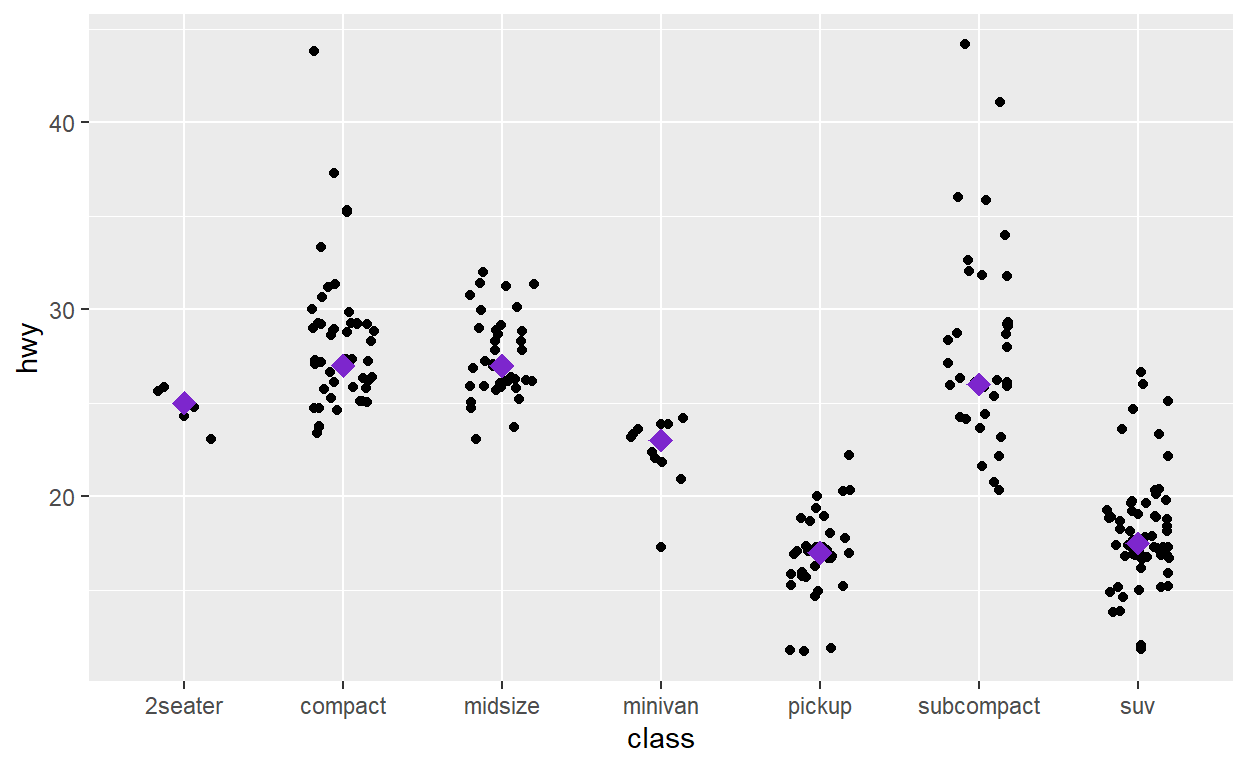# Exploratory analysis

Data visualization, part 1. Code for Quiz 7.

1. Load the R packages we will use
``````library(tidyverse)
``````

# Question: modify slide 34

• create a plot with the `faithful` dataset

• add point with `geom_point`

• assign the variable `eruptions` to the x-axis
• assign the variable `waiting` to the y-axis
• colour the points according to whether `waiting` is smaller or greater than 57
``````ggplot(faithful) +
geom_point(aes(x= eruptions, y= waiting, color = waiting > 57))
``````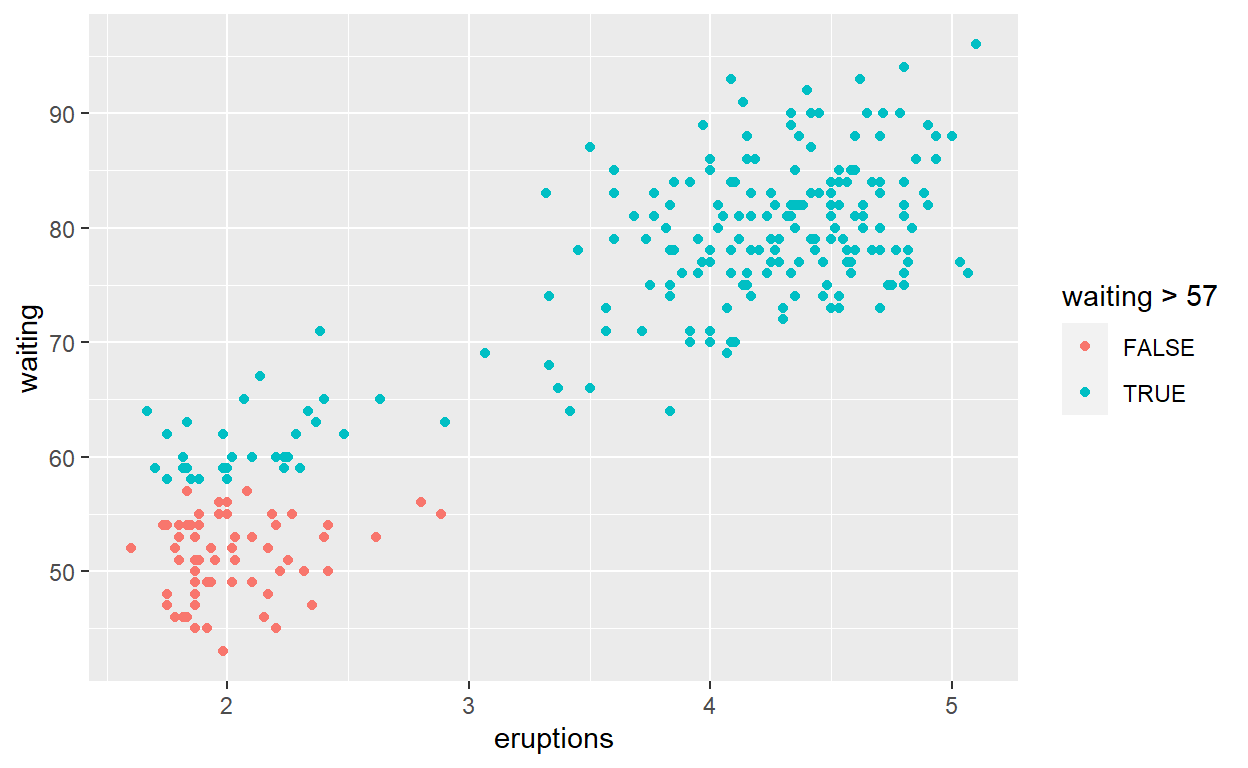# Question: modify intro-slide 35

• create a plot with `faithful` dataset

• add points with `geom_point`

• assign the variable `eruptions` to the x-axis
• assign the variable `waiting` to the y-axis
• assign the colour blueviolet to all the points
``````ggplot(faithful) +
geom_point(aes(x = eruptions, y = waiting),
colour = "blueviolet")
``````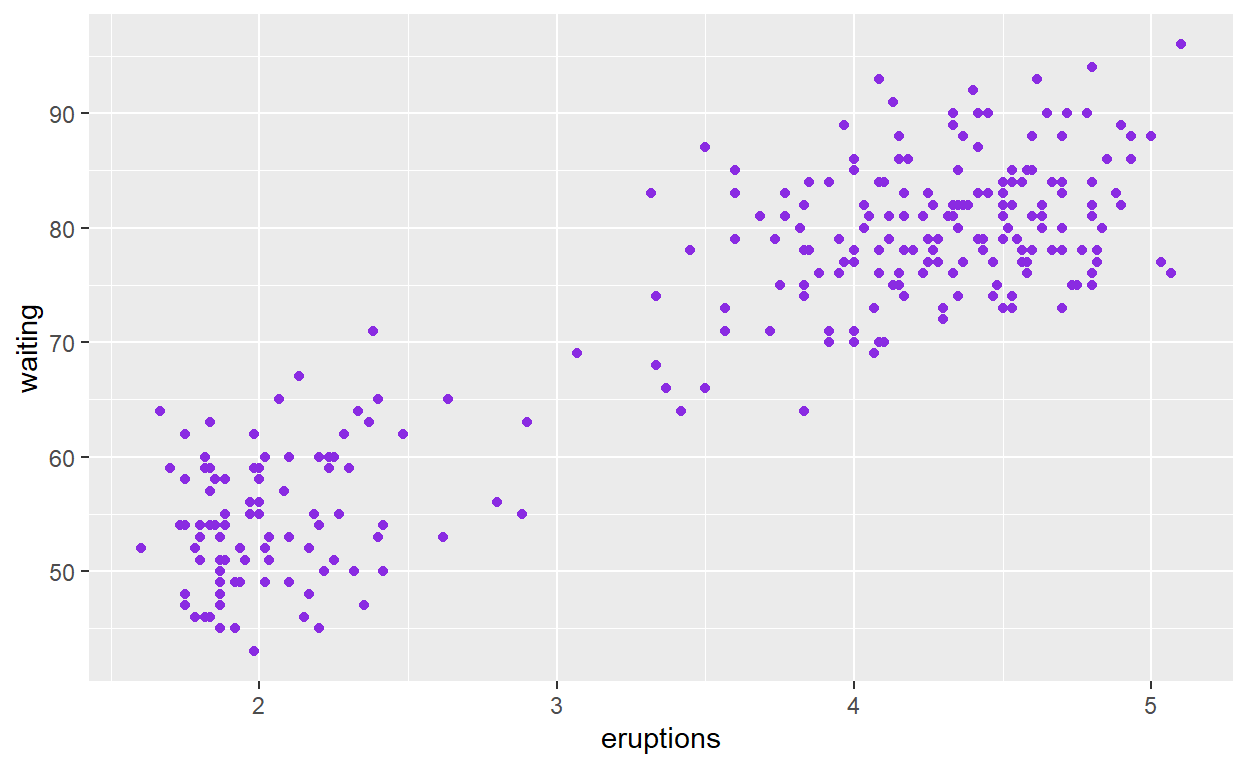# Question: Modify intro-slide 36

• create a plot with the `faithful` dataset

• use `geom_histogram()` to plot the distribution of `waiting` time

• assign the variable `waiting` to the x-axis
``````ggplot(faithful) +
geom_histogram(aes(x = waiting))
``````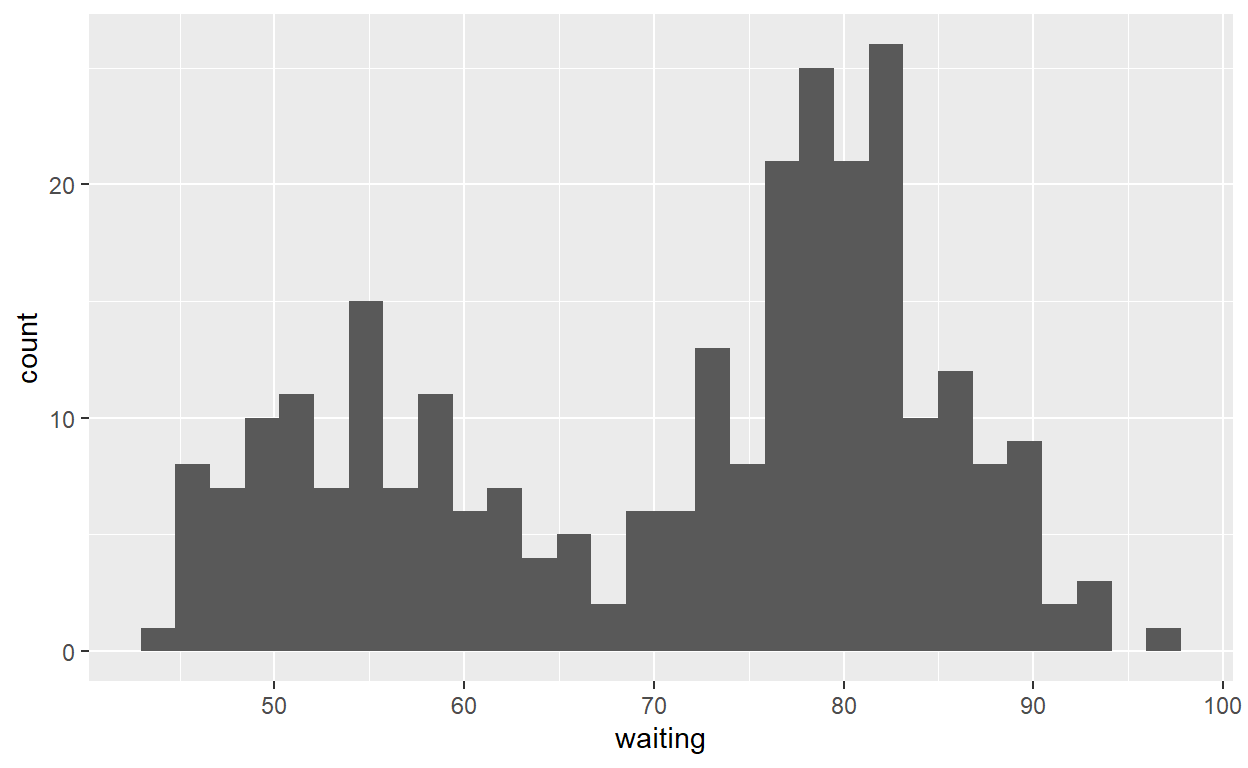# Question: Modify geom-ex-1

• see how shapes and sizes of points can be specified here: https://ggplot2.tidyverse.org/articles/ggplot2-specs.html#sec:shape-spec

• create a plot with the `faithful` dataset

• add points with `geom_point`

• assign the variable `eruptions` to the x-axis
• assign the variable `waiting` to the y-axis
• set shape of the points to plus
• set the point size to 1
• set the point transparency 0.4
``````ggplot(faithful) +
geom_point(aes(x = eruptions, y = waiting),
shape = "plus", size = 1, alpha = 0.4)
``````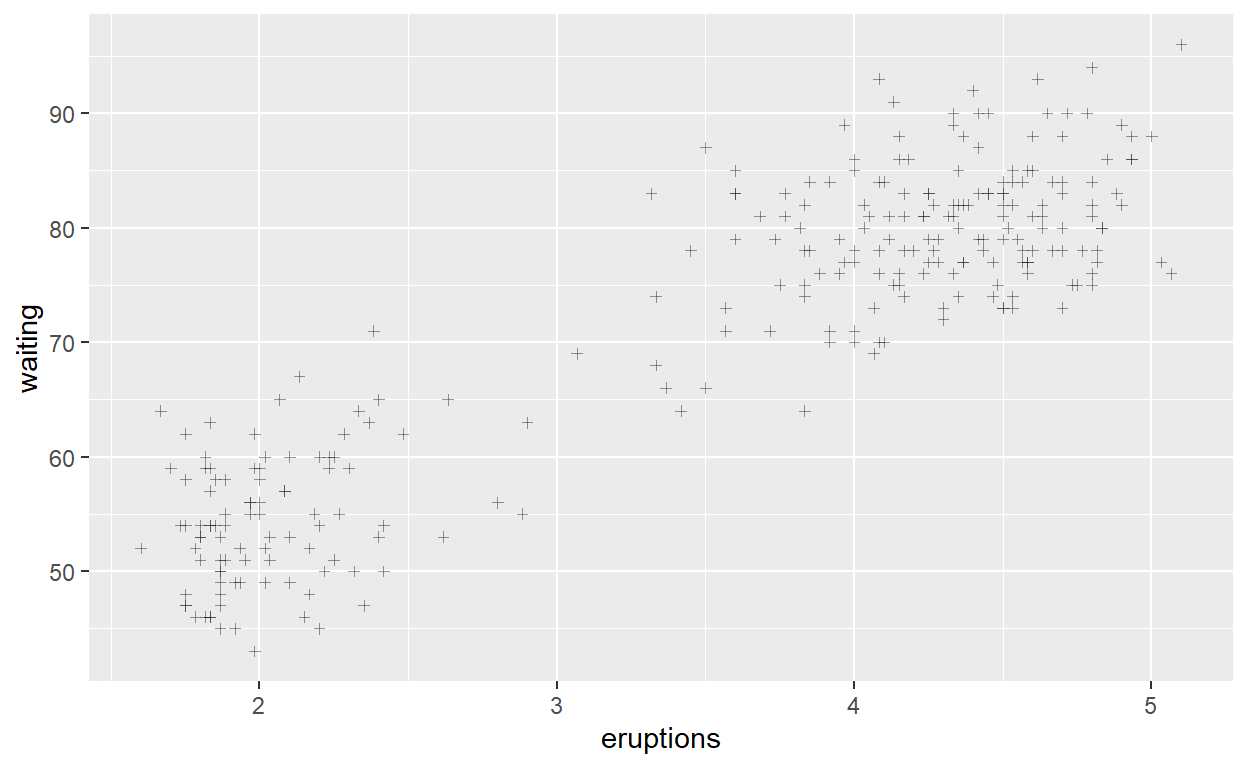# Question: Modify geom-ex-2

• Create a plot with the `faithful` dataset

• use `geom_histogram()` to plot the distribution of the `eruptions` (time)

• fill in the histogram based on whether eruptions are greater than or less than 3.2 minutes

``````ggplot(faithful) +
geom_histogram(aes(x = eruptions, fill = "time" > 3.2))
``````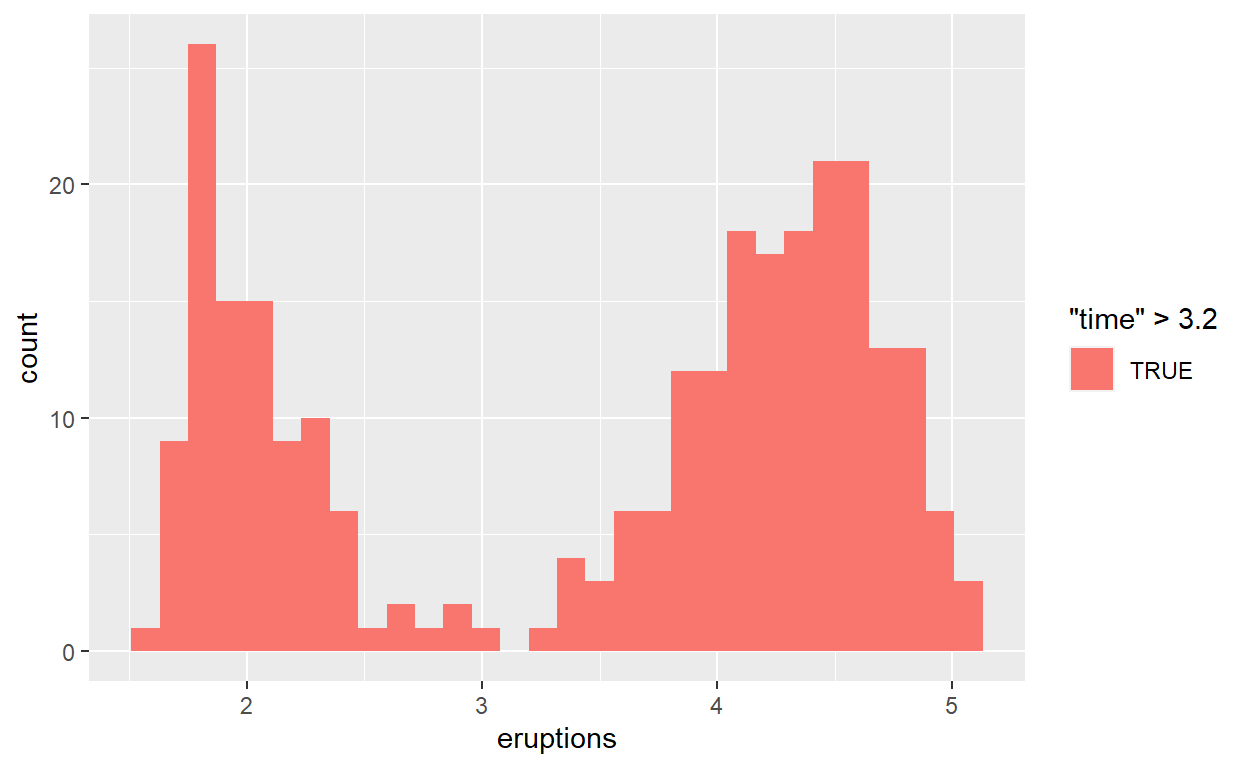# Question: Modify stat-slide-40

• create a plot with the `mpg` dataset

• add `geom_bar()` to create a bar chart of the variable `manufacturer`

``````ggplot(mpg) +
geom_bar(aes(x = manufacturer))
``````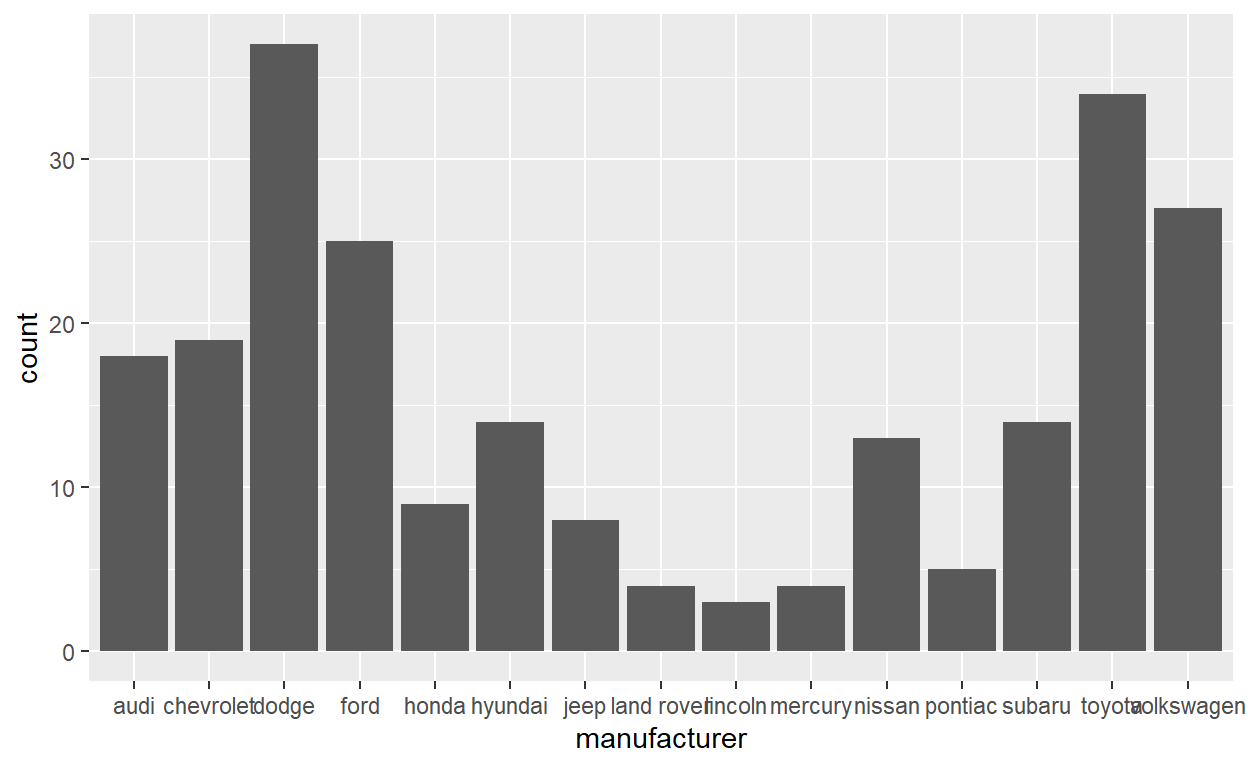# Question: Modify stat-slide-41

• change code to count and to plot the variable `manufacturer` instead of `class`
``````mpg_counted <- mpg %>%
count(manufacturer, name = 'count')
ggplot(mpg_counted) +
geom_bar(aes(x = manufacturer, y = count), stat = 'identity')
``````# Question: Modify stat-slide-43

• change the code to plot bar chart of each manufacturer as a percent of total

• change `class` to `manufacturer`

``````ggplot(mpg) +
geom_bar(aes(x = manufacturer, y = after_stat(100 * count / sum(count))))
``````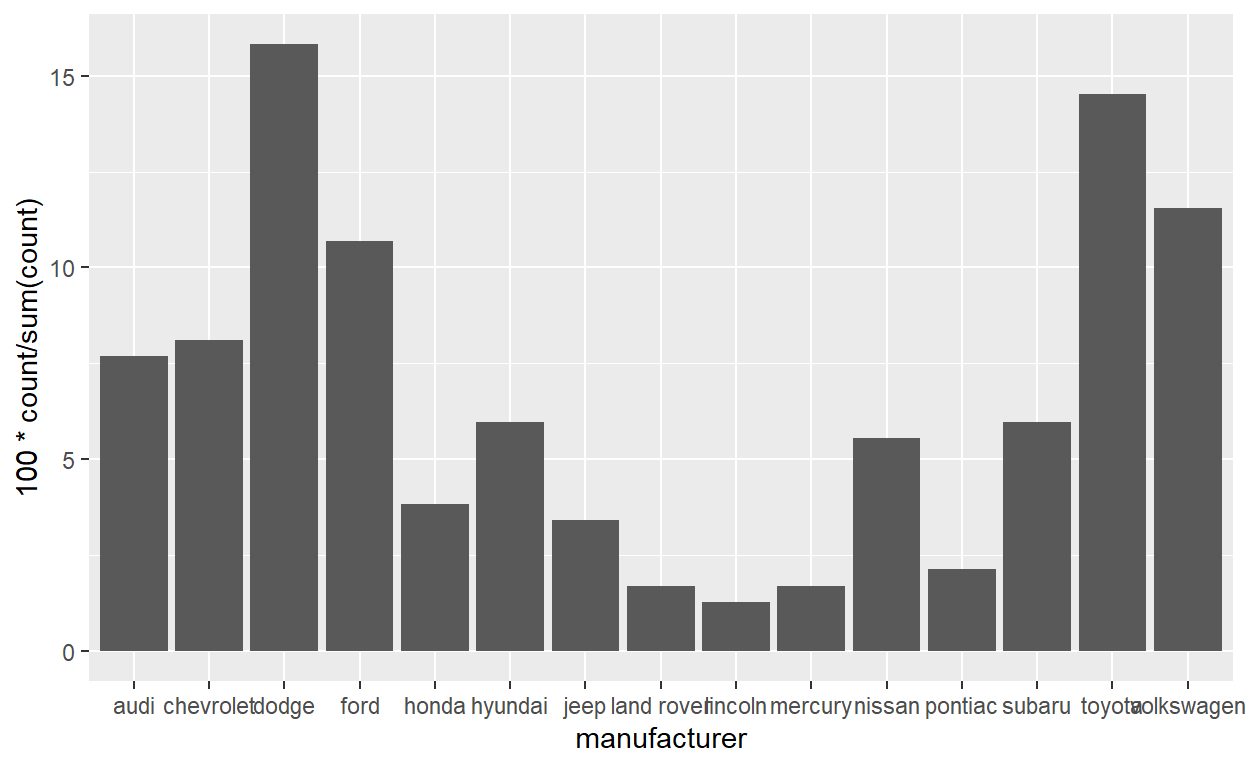# Question: Modify answer to stat-ex-2

• use `stat_summary()` to add a dot at the `median` of each group

• color the dot purple3
• make the shape of the dot diamond
• make the dot size 4
``````ggplot(mpg) +
geom_jitter(aes(x = class, y = hwy), width = 0.2) +
stat_summary(aes(x = class, y = hwy), geom = "point",
fun = "median", color = "purple3",
shape = "diamond", size = 4)
``````